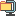검색어 입력폼

# DSP 매트랩 #2 이동 평균 필터

저작시기 2009.05 |등록일 2009.05.31압축파일 (zip) | 11페이지 | 가격 2,000원

## 소개글

m파일과 각 사진도 첨부하였습니다.

Homework (Matlab#2)

0. Ftp the data file `KTX` from the ftp site "ftp://iclab.ac.kr/DSP" to your folder.

In Matlab command window, load the data file "KTXSignal`. Check the data variable. The sampling rate of the signal is 100/sec.

1. Plot `KTXSignal` along the actual time axis `t`.
>> plot(t,signal)

2. Plot `KTXSignalWithNoise` along the actual time axis `t`.
>> plot(t,KTXSignalWithNoise)

3. We want to remove the noise from `SigWithNoise` by an average filter . We denote the filtered signal as . For an example, when L=10, in Matlab
>>L=10;
>>h=ones(1,L)/L;
>>y=conv(h,KTXSignalWithNoise);

1) Check for the length of y[n]. Let p be the length of y[n]. Determine the length of y[n] in terms of L and M=length(SigWithNoise).

2) When L=10,
● plot y[n] in discrete index n and also y in time scale.
>> plot(y);
>> plot(t,y); % length of t should be adjusted to the length of y.
● plot the frequency response of `h[n]`. In Matlab, you can use `freqz`
>> [Hf,theta]=freqz(h,1);
>> plot(theta,abs(H)); % for ploting magnitude of `h[n]`.
>> plot(theta,angle(H)); % for ploting angle of `h[n]`.

3) Repeat 2) for L=2,5,10,15,20. Observe the following as L changes.
● Degree of noise removing and smoothing y[n].
● The transient time.
● Determine the optimal L.
● Shape of abs(H(theta)). (sharpness of cutoff frequency, magnitude of side lobes)
=> You can overlay `abs(H(theta))` for L=2,10,20. Then you can recognize the shape change of abs(H(theta)).

매트랩

## 압축파일 내 파일목록

Movingavr.m
1-1.jpg
2-1.jpg
3-1.jpg
3-2.jpg
3-3.jpg
3-4.jpg
3-5 L=2.jpg
3-6 L=5.jpg
3-7 L=10.jpg
3-8 L=15.jpg
3-8 L=20.jpg
3-9 impulseresp.jpg
참고사항.hwp
HW2noisesignal.m
HW2nomalsignal.m
HW31.m
ImpulseResp.m
KTXSignal.mat
KTXSignalWithNoise.mat
Matlab(HW2).hwp
MatlabHW.mat

없음